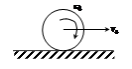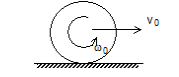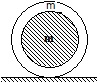## Filters

Sort by :
Clear All
Q
Engineering
76 Views   |

A cylinder of mass Mc and sphere of mass Ms are placed at points A and B of two  inclines, respectively.

(See Figure). If they roll on the incline without slipping such that their accelerations are the same, then

The ratiois• Option 1)• Option 2)• Option 3)• Option 4)As we have learned Rolling of a body on an inclined plane - - wherein K=Radius of gyration = Angle of inclination      Acceleration along inclined plane  FOr sphere    For cylinder          Option 1) Option 2) Option 3) Option 4)
Engineering
597 Views   |

When a steady torque is acting on a body, the body

• Option 1)

Continues in its state of rest or uniform motion along a straight line

• Option 2)

Gets linear acceleration

• Option 3)

Gets angular acceleration

• Option 4)

Rotates at a constant speed

As we learnt in  Torque -       - wherein This can be calculated by using either  = perpendicular distance from origin to the line of force. = component of force perpendicular to line joining force.    The body rotates at a constant speed. Option 1) Continues in its state of rest or uniform motion along a straight line incorrect Option 2) Gets linear acceleration incorrect Option 3) Gets...
Engineering
103 Views   |

When a mass is rotating in a plane about a fixed point its angular momentum is directed along.

• Option 1)

• Option 2)

The tangent to orbit

• Option 3)

The line at an angle of 45° to the plane of rotation

• Option 4)

The axis of rotation

As we learnt in Centre of Mass of semicircular ring - It lies at a distance of  from centre of the ring along its axis. -    Along axis of rotation. Option 1) The radius Incorrect Option 2) The tangent to orbit Incorrect Option 3) The line at an angle of 45° to the plane of rotation Incorrect Option 4) The axis of rotation Correct
Engineering
202 Views   |

Two disc with same mass but different radii are moving with same K.E. One of them rolls and other slides without friction. Then

• Option 1)

Rolling disc has greater velocity

• Option 2)

Sliding disc has greater velocity

• Option 3)

Both have same velocity

• Option 4)

The disc with greater radius will have greater velocity

As we learnt in  Rolling of a body on an inclined plane - - wherein K=Radius of gyration = Angle of inclination     Sliding disc has greater velocity. Option 1) Rolling disc has greater velocity Incorrect Option 2) Sliding disc has greater velocity correct Option 3) Both have same velocity Incorrect Option 4) The disc with greater radius will have greater velocity Incorrect
Engineering
94 Views   |

The torque acting is 2000 Nm with an angular acceleration of 2 rad/. The moment of inertia of body is

• Option 1)

1200 kg

• Option 2)

900 kg

• Option 3)

1000 kg

• Option 4)

Can't say

As we learnt in  Analogue of second law of motion for pure rotation - - wherein Torque equation can be applied only about two point (i) centre of motion. (ii) point which has zero velocity/acceleration.       Option 1) 1200 kg  Incorrect Option 2) 900 kg  Incorrect Option 3) 1000 kg  correct Option 4) Can't say Incorrect
Engineering
87 Views   |

The term moment of momentum is called

• Option 1)

Momentum

• Option 2)

Force

• Option 3)

Torque

• Option 4)

Angular Momentum

Angular momentum - - wherein   represent angular momentum of a moving particle about a point. it can be calculated  as = Length of perpendicular on line of motion = component of momentum along perpendicualar to     Option 1) Momentum Incorrect Option 2) Force Incorrect Option 3) Torque Incorrect Option 4) Angular Momentum correct
Engineering
831 Views   |

The speed of a homogeneous, solid sphere after rolling down in the inclined plane of vertical height h, from rest without sliding is

• Option 1)

• Option 2)

• Option 3)

• Option 4)

As we learnt in  Rolling of a body on an inclined plane - - wherein K=Radius of gyration = Angle of inclination         Option 1) correct Option 2) Incorrect Option 3) Incorrect Option 4) Incorrect
Engineering
168 Views   |

The moment of inertia of a circular ring about an axis passing through its centre and normal to its plane is 200 gm , then its moment of inertia about a diameter is

• Option 1)

400 gm

• Option 2)

300 gm

• Option 3)

200 gm

• Option 4)

100 gm

As we learnt in Perpendicular Axis theorem - (for a body in XY plane ) - wherein = moment of inertia about z axis . :moment of inertia about x & y  axis in the plane of body respectively.     Option 1) 400 gm  This option is incorrect. Option 2) 300 gm  This option is incorrect. Option 3) 200 gm  This option is incorrect. Option 4) 100 gm  This option is correct.
Engineering
89 Views   |

The moment of inertia of a body comes into play

• Option 1)

In motion along a curved path

• Option 2)

In linear motion

• Option 3)

In rotational motion

• Option 4)

None of the above

As we learnt in Moment of inertia - Moment of inertia  (I) of a body is a measure of its ability to resist change in its rotational state of motion. - wherein Moment of inertia play the same role in rotatory motion as is played by mass in translatory motion .     The moment of inertia of a body comes into play in rotational motion. Option 1) In motion along a curved path This option is...
Engineering
191 Views   |

The M.I. of a solid cylinder of mass M and radius R about a line parallel to the axis of the cylinder and ling on the surface of the cylinder is

• Option 1)

• Option 2)

• Option 3)

• Option 4)

As we learnt in Paraller Axis Theorem - - wherein  is axis parallel to & an axis passing through centre of mass.       Option 1) This option is incorrect. Option 2) This option is incorrect. Option 3) This option is correct. Option 4) This option is incorrect.
Engineering
160 Views   |

If I, a and t are the moment of inertia, angular acceleration and torque respectively of a body rotating about any axis with angular velocity w, then

• Option 1)

t = Ia

• Option 2)

t = Iw

• Option 3)

I = tw

• Option 4)

a = Iw

As we learnt in Analogue of second law of motion for pure rotation - - wherein Torque equation can be applied only about two point (i) centre of motion. (ii) point which has zero velocity/acceleration.     Option 1) t = Ia This option is correct. Option 2) t = Iw This option is incorrect. Option 3) I = tw This option is incorrect. Option 4) a = Iw This option is incorrect.
Engineering
441 Views   |

By keeping moment of inertia of a body is constant, if we double the time period, then angular momentum of body is

• Option 1)

Remains constant

• Option 2)

Doubles

• Option 3)

Becomes half

• Option 4)

As we learnt in

Law of conservation of angular moment -

- wherein

If net torque is zero

i.e.

angular momentum is conserved only when external torque is zero .

Becomes half.

Option 1)

Remains constant

Incorrect

Option 2)

Doubles

Incorrect

Option 3)

Becomes half

Correct

Option 4)

Incorrect

View More
Engineering
515 Views   |

A wheel of mass 2 kg having practically all the mass concentrated along the circumference of a circle of radius 20 cm, is rotating on its axis with an angular velocity of 100 rad/s. The rotational kinetic energy of the wheel is

• Option 1)

4 J

• Option 2)

70 J

• Option 3)

400 J

• Option 4)

800 J

As we learnt in Kinetic energy of rotation - - wherein = moment of inertia about axis of rotation = angular velocity     Option 1) 4 J This solution is incorrect  Option 2) 70 J  This solution is incorrect  Option 3) 400 J This solution is correct  Option 4) 800 J This solution is incorrect
Engineering
108 Views   |

A uniform solid circular cylinder of radius r is placed on a rough horizontal surface and given a linear velocity  and angular velocity  as shown in the figure. The speed of cylinder when it starts rolling• Option 1)

5/2

• Option 2)

3/2

• Option 3)

5/3

• Option 4)

2/3

As we learnt in Law of conservation of angular moment - - wherein If net torque is zero i.e. angular momentum is conserved only when external torque is zero .   Whence  Option 1) 5/2 This solution is incorrect  Option 2) 3/2  This solution is incorrect  Option 3) 5/3  This solution is correct  Option 4) 2/3  This solution is incorrect
Engineering
123 Views   |

The moment of inertia of a disc about its geometrical axis is I. then its M.I. about its diameter will be

• Option 1)

I

• Option 2)

2I

• Option 3)

• Option 4)

Moment of inertia for system of particle -   - wherein Applied when masses are placed discretely.     Option 1) I Incorrect Option 2) 2I Incorrect Option 3) correct Option 4) Incorrect
Engineering
404 Views   |

A uniform disc of mass 2 kg is rotated about an axis perpendicular to the plane of the disc. If radius of gyration is 50 cm, then the M.I. of disc about same axis is

• Option 1)

0.25 kg

• Option 2)

0.5 kg

• Option 3)

2 kg

• Option 4)

1 kg

As we discussed in Radius of gyration (K) - Radius of Gyration of a body about an axis is the effective distance from the axis where the whole mass can be assumed to be concentrated so that moment of inertia remains the same. - wherein    use I=MK2 Option 1) 0.25 kg  This solution is incorrect  Option 2) 0.5 kg  This solution is correct  Option 3) 2 kg  This solution is incorrect  Option...
Engineering
89 Views   |

A uniform circular disc of radius r is placed on a rough horizontal surface and given a linear velocity vo and angular velocity  as shown. The disc comes to rest after moving some distance to the right. It follows that• Option 1)

• Option 2)

• Option 3)

• Option 4)

As we discussed concept Law of conservation of angular moment - - wherein If net torque is zero i.e. angular momentum is conserved only when external torque is zero .    Angular momentum about P is conserved Option 1) This is incorrect option Option 2) This is correct option Option 3) This is incorrect option Option 4) This is incorrect option
Engineering
244 Views   |

A thin uniform, circular ring is rolling down an inclined plane of inclination 30° without slipping. Its linear acceleration along the inclined plane will be

• Option 1)

g/2

• Option 2)

g/3

• Option 3)

g/4

• Option 4)

2g/3

As we discussed in Rolling of a body on an inclined plane - - wherein K=Radius of gyration = Angle of inclination       Option 1) g/2 This is incorrect option Option 2) g/3 This is incorrect option Option 3) g/4 This is correct option Option 4) 2g/3 This is incorrect option
Engineering
817 Views   |

A thin hollow sphere of mass m is completely filled with an ideal liquid of mass m. When the sphere rolls with a velocity v kinetic energy of the system is equal to• Option 1)

(1/2)

• Option 2)

• Option 3)

(4/3)

• Option 4)

(4/5)

As we discussed in concept Kinetic energy of rotation - - wherein = moment of inertia about axis of rotation = angular velocity     K.E of the system Option 1) (1/2)  This is incorrect option Option 2) This is incorrect option Option 3) (4/3)  This is correct option Option 4) (4/5)  This is incorrect option
Engineering
174 Views   |

A thin circular ring of mass M and radius R is rotating about its axis with a constant angular velocity ω . Two objects, each of mass m are attached gently to the opposite ends of the diameter of the ring. The wheel now rotates with an angular velocity.

• Option 1)

ω M/(M + m)

• Option 2)

{(M – 2m)/(M +2m)}ω

• Option 3)

{M/(M + 2m)}ω

• Option 4)

{(M + 2m)/M} ω

As we discussed in concept Law of conservation of angular moment - - wherein If net torque is zero i.e. angular momentum is conserved only when external torque is zero .       Option 1) ω M/(M + m) This is incorrect option Option 2) {(M – 2m)/(M +2m)}ω This is incorrect option Option 3) {M/(M + 2m)}ω This is correct option Option 4) {(M + 2m)/M} ω This is incorrect option
Exams
Articles
Questions